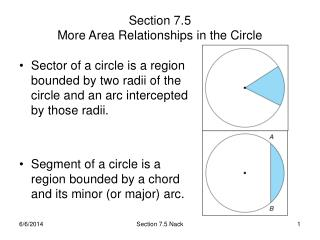Download PresentationSection 7.5 More Area Relationships in the Circle

# Section 7.5 More Area Relationships in the Circle - PowerPoint PPT PresentationDownload Presentation## Section 7.5 More Area Relationships in the Circle

- - - - - - - - - - - - - - - - - - - - - - - - - - - E N D - - - - - - - - - - - - - - - - - - - - - - - - - - -
##### Presentation Transcript

1. Sector of a circle is a region bounded by two radii of the circle and an arc intercepted by those radii. Segment of a circle is a region bounded by a chord and its minor (or major) arc. Section 7.5More Area Relationships in the Circle Section 7.5 Nack

2. Area of a Sector • Postulate 23: The ratio of the degree measure m of the arc (or central angle) of a sector to 360 is the same as the ratio of the area of the sector to the area of the circle: area of sector = m area of circle 360 • Theorem 7.5.1: In a circle of radius r, the area A of a sector whose arc has degree measure m is given by: A = m r² 360 • Corollary 7.5.2: The area of a semicircular region of radius r is A = ½r² Example 1-3 p. 373-4 Section 7.5 Nack

3. Area of a Segment • Area of a Segment is the area of the sector minus the area of the triangle formed using the center of the circle and the endpoints of the sector. Asegment = Asector – AΔ Asegment = 90 12² - ½ 1212 360 =(36 - 72) square units. 12 12 Section 7.5 Nack

4. Area of a Triangle with an inscribed circle • Theorem 7.5.3: Where P represents the perimeter of a triangle and r represents the length of the radius of its inscribed circle, the area of the triangle is given by: A = ½rP Section 7.5 Nack